# Methods and formulas for Test for Equal Variances

Select the method or formula of your choice.

## Multiple comparisons test

### Multiple comparison intervals for k > 2 samples

Let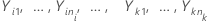be k > 2 independent samples, each sample being independent and identically distributed with mean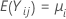and variance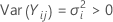. And, suppose that the samples originate from populations that have a common kurtosis.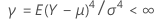And let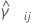be a pooled kurtosis estimator for the pair of samples ( i, j) given as:

Let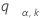be the upper a point of the range of k variables that are independent and identically distributed on a standard normal random distribution. That is,satisfies the following:

where Z1, ..., Zk are independent and identically distributed standard normal random variables. Barnard (1978) provides a simple numerical algorithm based on a 16-point Gaussian quadrature for calculating the distribution function of the normal range.

The multiple comparison procedure rejects the null hypothesis of equality of variances (also called homogeneity of variances) if, and only if, at least one pair of the following intervals do not overlap:

where

where ri = (ni - 3) / ni.

We refer to the above intervals as multiple comparison intervals or MC intervals. The MC intervals for each sample are not to be interpreted as confidence intervals for the standard deviations of the parent populations. Hochberg et al. (1982) refer to similar intervals for comparing means as "uncertainty intervals". The MC intervals given here are useful only for comparing the standard deviations or variances for multi-sample designs. When the overall multiple comparison test is significant, the standard deviations that correspond to the non-overlapping intervals are statistically different. (For the detailed derivation of these intervals, go to the white paper on Multiple comparison methods.)

### Multiple comparison intervals for k = 2 samples

When there are only two samples, the multiple comparison intervals are given by:

where zα / 2 is the upper α / 2 percentile point of the standard normal distribution, ci = ni / ni - zα / 2, and Vi is given by the following formula:

### P-value for the test

If there are 2 samples in the design, then Minitab calculates the p-value for the multiple comparisons test using Bonett's method for a 2 variances test and a hypothesized ratio, Ρo, of 1.

If there are k > 2 samples in the design, then let Pi j be the p-value of the test for any pair (i, j ) of samples. The p-value for the multiple comparisons procedure as an overall test of equality of variances is given by the following:

For more information, including simulations and detailed algorithms for calculating Pi j , go to Bonett's Method, which is a white paper that has simulations and other information about Bonett's Method.

### Notation

TermDescription
nithe number of observations in sample i
Y i l the lth observation in sample i
mithe trimmed mean for sample i with trim proportions of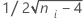kthe number of samples
Sithe standard deviation of sample i
αthe significance level for the test = 1 - (the confidence level / 100)
Ci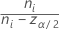Zα / 2the upper α / 2 percentile point of the standard normal distribution
ri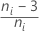## Levene's test statistic

Minitab displays a test statistic and a p-value for Levene's test. The null hypothesis is that the variances are equal, and the alternative hypothesis is that the variances are not equal. Use Levene's test when the data come from continuous, but not necessarily normal, distributions.

The calculation method for Levene's test is a modification of Levene's procedure (Levene, 1960) that was developed by Brown and Forsythe (1974). This method considers the distances of the observations from their sample median rather than their sample mean. Using the sample median rather than from the sample mean makes the test more robust for smaller samples and makes the procedure asymptotically distribution-free. If the p-value is smaller than your α-level, reject the null hypothesis that the variances are equal.

### Formula

• H. Levene (1960). Contributions to Probability and Statistics. Stanford University Press, CA.
• M. B. Brown and A. B. Forsythe (1974). "Robust tests for the equality of variance," Journal of the American Statistical Association, 69, 364-367.

### Notation

TermDescription
Vij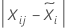i1, ..., k
j1, ..., ni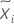median

## Bartlett's test statistic

Minitab displays a test statistic and a p-value for Bartlett's test. When there are only two levels, Minitab performs an F-test instead of Bartlett's test. For these tests, the null hypothesis is that the variances are equal, and the alternative hypothesis is that the variances are not equal. Use Bartlett's test when the data are from normal distributions; Bartlett's test is not robust to departures from normality.

Bartlett's test statistic calculates the weighted arithmetic average and weighted geometric average of each sample variance based on the degrees of freedom. The greater the difference in the averages, the more likely the variances of the samples are not equal. B follows a χ2 distribution with k – 1 degrees of freedom. If the p-value is smaller than your α-level, reject the null hypothesis that the variances are equal.

### Notation

TermDescription
si2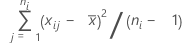knumber of samples
vini - 1
ninumber of observations at the ith factor level

## F-test statistic

When there are only two levels, Minitab performs an F-test instead of Bartlett's test. The null hypothesis is that the variances are equal, and the alternative hypothesis is that the variances are not equal. Use the F-statistic when the data are from normal distributions.

If the p-value is less than the α-level, reject the null hypothesis that the variances are equal.

### Notation

TermDescription
S12variance of sample 1
S22variance of sample 2
n1 - 1degrees of freedom for numerator
n2 - 1degrees of freedom for denominator

## Standard deviation (StDev)

### Notation

TermDescription
yijobservations at the ith factor level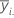mean of observations at the ith factor level
ninumber of observations at the ith factor level

## Bonferroni confidence intervals

Minitab calculates confidence intervals for the standard deviations using the Bonferroni method. A confidence interval is a range of values that is likely to include some population parameter, in this case, contain the standard deviation.

Standard confidence intervals are calculated using a confidence level 1 – α / 2. the Bonferroni method uses the confidence level 1 – α / 2p for each individual confidence interval, where p is the number of factor and level combinations. The method ensures that the set of confidence intervals has a confidence level of at least 1 – α. The Bonferroni method provides more conservative (wider) confidence intervals, which reduces the probability of a type 1 error.

By using this site you agree to the use of cookies for analytics and personalized content.  Read our policy